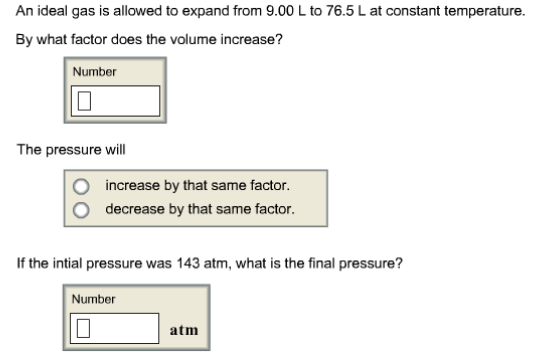# Problem: An ideal gas is allowed to expand from 9.00 L to 76.5 L at constant temperature. By what factor does the volume increase? The pressure will                  • increase by that same factor                   • decrease by that same factor If the intial pressure was 143 atm, what is the final pressure?

###### FREE Expert Solution
84% (370 ratings)###### Problem Details

An ideal gas is allowed to expand from 9.00 L to 76.5 L at constant temperature.

By what factor does the volume increase?

The pressure will

• increase by that same factor

• decrease by that same factor

If the intial pressure was 143 atm, what is the final pressure?